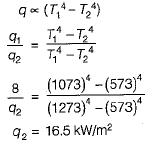Courses

# Test: Radiation Heat Transfer - 3

## 30 Questions MCQ Test Topicwise Question Bank for Mechanical Engineering | Test: Radiation Heat Transfer - 3

Description
This mock test of Test: Radiation Heat Transfer - 3 for Chemical Engineering helps you for every Chemical Engineering entrance exam. This contains 30 Multiple Choice Questions for Chemical Engineering Test: Radiation Heat Transfer - 3 (mcq) to study with solutions a complete question bank. The solved questions answers in this Test: Radiation Heat Transfer - 3 quiz give you a good mix of easy questions and tough questions. Chemical Engineering students definitely take this Test: Radiation Heat Transfer - 3 exercise for a better result in the exam. You can find other Test: Radiation Heat Transfer - 3 extra questions, long questions & short questions for Chemical Engineering on EduRev as well by searching above.
QUESTION: 1

### According to Stefan Boltzmann law, the total radiation from a black body per second per unit area is proportional to

Solution:

Stefen boltzman law is given by
Q = σAT4
hence Q ∝ T4

QUESTION: 2

Solution:
QUESTION: 3

### Absorptivity of a body is equal to its emissivity

Solution:
QUESTION: 4

The ratio of total emissive power of body to the total emissive power of a black body at the same temperature is called

Solution:

Emissivity for any body is given by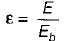Where
E = Emissive power of a body
Eb = Emissive power of a black body

QUESTION: 5

Which one of the following is true for black body

Solution:

Since black body is perfect absorber of radiation hence absorptivity of black body α = 1.

QUESTION: 6

Which one of the following is true for white body?

Solution:
QUESTION: 7

A surface is called Grey surface. If

Solution:
QUESTION: 8

The unit of Stefan-Botlzmann constant is

Solution:
QUESTION: 9

When metallic surfaces are oxidized, the emissivity

Solution:
QUESTION: 10

The solar energy falling on the earth’s surface is called

Solution:
QUESTION: 11

The shape factor F12 in case of a conical cavity having a semi vertex angle a and height h is given by

Solution: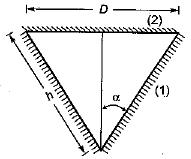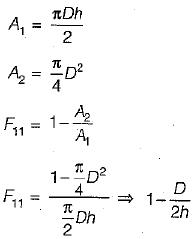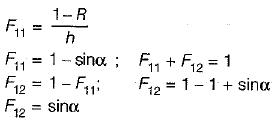QUESTION: 12

If the temperature of a solid surface changes from 27°C to 627°C. Its emissive power will increase in the ratio of

Solution:

Since emissive power = f(T4)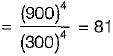QUESTION: 13

The radiative heat transfer rate per unit area (W/m2) between two plane parallel grey surface (emissivity = 0.9) maintained at 400 K and 300 K is (StefanBoltzmann constant s = 5.67 x 10-8 W/m2K4)

Solution: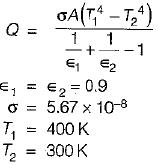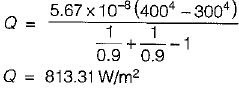QUESTION: 14

Two long parallel surfaces each of emissivity 07 are maintained at different temperature and accordingly have radiation heat exchange between them. It is desirable to reduce 75% of this radiant heat transfer by inserting thin parallel shields of equal emissivity on both sides. The number of shields should be

Solution: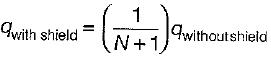where N = Number of radiation shield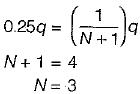QUESTION: 15

Heat transfer by radiation between two gre bodies of emissivity ∈ is proportional to (Notations have their usual meanings)

Solution:

(α = ∈) Kirchoff’s law
q = A- αiGi)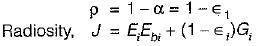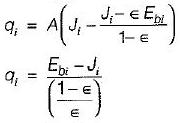QUESTION: 16

The shape factor of a hemispherical body place on a flat surface with respect to it self is

Solution: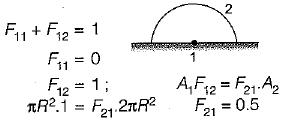QUESTION: 17

The shape factor of a cylindrical cavity with respect to it self is

Solution: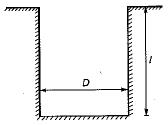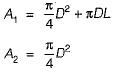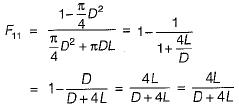QUESTION: 18

A spherical body with surface A1 is completely enclosed by another hollow body with inner surface A2. The shape factor of A2 with respect to A1 is

Solution:
QUESTION: 19

For a circular tube of equal length and diameter shown below, the view factor F13 = 0.17. The view factor F12 in this case will be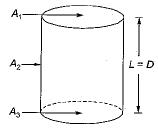Solution:

f11 + f12 + f13 = 1
f11 = 0
f12 = 1 - f13
f12 = 1 - 0.17 = 0.83

QUESTION: 20

For a hemisphere, the solid angle is measured

Solution:
QUESTION: 21

Two spheres A and B of same material have radii 1m and 4m and temperature 1000 K and 2000 K respectively. Which one of the following statement is correct.
The energy radiated by sphere A is

Solution: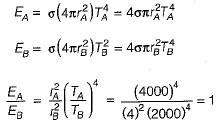QUESTION: 22

A plate having 10 cm2 area each side is hanging in the middle of a room of 100 m2 total surface area, the plate temperature and emissivity are respectively 800 K and 0.6. The temperature and emissivity values for the surface of the room are 300 K and 0.3 respectively. Boltzman’ constant σ = 5.67 x 10-8 W/m2K4. The total heat loss from the two surface of the plate is

Solution:

A1 = 20 cm2 = 20 x 10-4m2
A= 100 m2
T1 = 800K
1 = 0.6
T= 300 K
2 = 0.3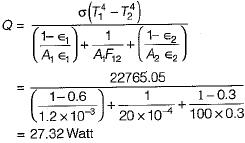QUESTION: 23

Match List-I (surface with orientations) with List-lI (equivalent emissivity) and select the correct answer using the codes given below:
List-I
A. Infinite parallel planes
B. Body 1 completely enclosed by body 2 but body 1 is very small
C. Radiation exchange between two small Grey bodies
D. Two concentric cylinders with large lengths
List-II
1. ∈1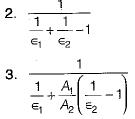4. ∈12
Codes:
A  B  C  D
(a)  3  1  4   2
(b)  2  4  1   3
(c)  2  1  4   3
(d)  3  4  1   2

Solution:
QUESTION: 24

For the two long concentric cylinders with surface areas A1, and A2, the view factor F22 is given by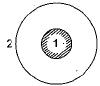Solution: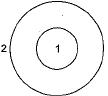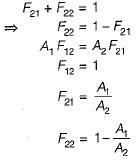QUESTION: 25

For a thin steel sheet, total emissive power is given as 32 W/m2, insolation as 93 W/m2. If thin sheet has reflectivity = 0,6, absorbtivity = 0.1 and transmissivity = 0.3. Then radiosity in W/m2

Solution:

Radiosity = J = E + ρG
J = 32 + 0.6(93)
J = 87.8 W.m-2

QUESTION: 26

The thermal radiative flux from a surface of emissivity = 0 .4 is 22.68 kW /m2. The approximate surface temperature (K) is
Stefan Boltzmann's constant = 5.67x10-8 W/m2K4

Solution:

We know, for radiation heat transfer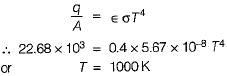QUESTION: 27

A solid cylinder (surface 2) is located at the centre of a hollow sphere (surface 1). The diameter of the sphere is 1 m, while the cylinder has a diameter and length of 0.5 m each. The radiation configuration factor F11 is

Solution:
QUESTION: 28

Match List-I (Thermal spectrum) with List-l! (Wavelength mm) and select the correct aswer using the code given below the lists:
List-I
A. Cosmic rays
C. Visible light
D. UVrays
List-II
1. λ < 10-8
2. 102 < λ < 1010
3. 0.38 < λ< 0.78
4. 10-2 < λ < 0.38
Codes:
A  B  C  D
(a) 4  2  3  1
(b) 2  1  3  4
(c) 4  3  2  1
(d) 1  2  3  4

Solution:
QUESTION: 29

Match List-I with List-ll and select the correct answer using the code given below the lists:
List-I
A. Window glass
B. Grey surface
C. Carbon dioxide
List-ll
1. Emissivity
2. Kinematic viscosity
3. Diffusion coefficient
Codes:
A  B  C
(a) 2  3  1
(b) 3  2  1
(c) 1  3  2
(d) 1  2  3

Solution:
QUESTION: 30

The radiation heat flux from a heating element at a temperature of 800°C, in a furnace maintained at 300°C is kW/m2. The flux when the element temperature is increased to 1000°C for the same furnace temperature is

Solution: